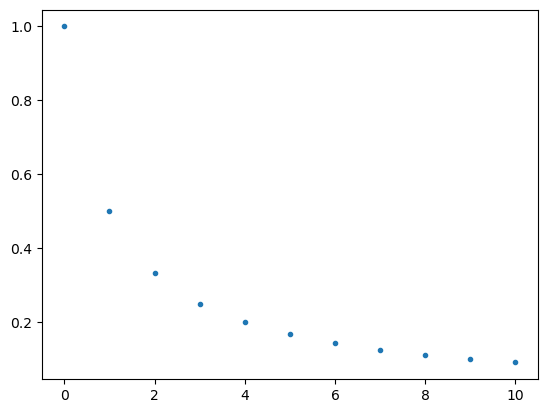# How to graph mathematical sequences (in Python, using SymPy and Matplotlib)

See all solutions.

Assume we have a mathematical sequence $a_0,a_1,a_2,\ldots$ and we would like to plot a graph of it using the standard Cartesian coordinate system. The result will not look like a curve, because a sequence is separate points instead of a smooth curve.

## Solution

This answer assumes you have imported SymPy as follows.

1
2
from sympy import *                   # load all math functions
init_printing( use_latex='mathjax' )  # use pretty math output


We will re-use the sequence defined in the task how to define a mathematical sequence.

1
2
3
4
var( 'n' )
a_n = 1 / ( n + 1 )
seq = sequence( a_n, (n,0,oo) )
seq


$\displaystyle \left[1, \frac{1}{2}, \frac{1}{3}, \frac{1}{4}, \ldots\right]$

We can graph any finite range of any sequence as follows.

1
2
3
4
5
start = 0
stop = 10
import matplotlib.pyplot as plt
plt.plot( range(start,stop+1), seq[start:stop+1], '.' )
plt.show()